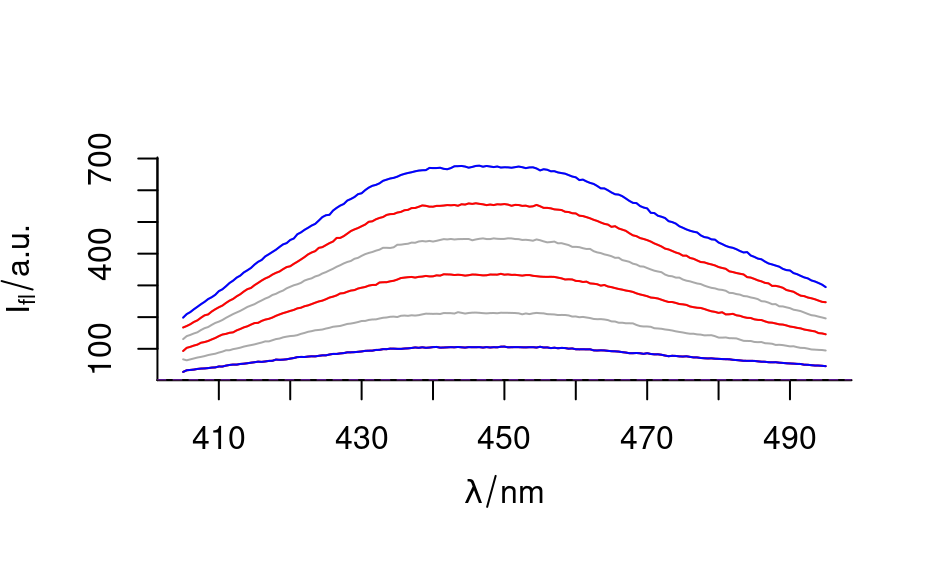This function generates sequences along the spectra (rows) or wavelengths of hyperSpec objects.

# S3 method for hyperSpec
seq(x, from = 1, to = nrow(x), ..., index = FALSE)

## Arguments

x

the hyperSpec object

from, to

arguments handed to base::seq.int()

...

arguments for base::seq(), namely by, length.out

index

should a vector with indices be returned rather than a hyperSpec object?

## Value

a numeric or hyperSpec object, depending on index.

## Details

Note that wl2i() generates sequences of indices along the wavelength axis.

seq() had to be implemented as S3 method as the generic has only ... arguments (on which no dispatch with differing types is possible).

base::seq_along() is not generic, but returns a sequence of the length of the object. As hyperSpec provides a Method length(), it can be used. The result is a sequence of indices for the spectra.

wl2i() to construct sequences of wavelength indices.

base::seq()

C. Beleites

## Examples


seq(flu, index = TRUE)
#>  1 2 3 4 5 6
seq_along(flu)
#>  1 2 3 4 5 6
seq(flu, length.out = 3, index = TRUE) # return value is numeric, not integer!
#>  1.0 3.5 6.0
seq(flu, by = 2, index = TRUE) # return value is numeric, not integer!
#>  1 3 5

plot(flu, col = "darkgray")
plot(seq(flu, by = 2), add = TRUE, col = "red")
plot(seq(flu, length.out = 2), add = TRUE, col = "blue")### needs to be an S3 function as S4 ... dispatch has to have the same
### signature for all parameters